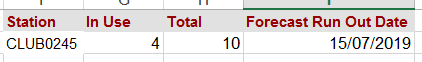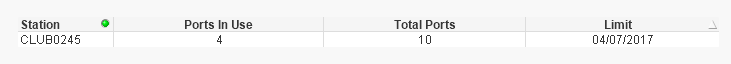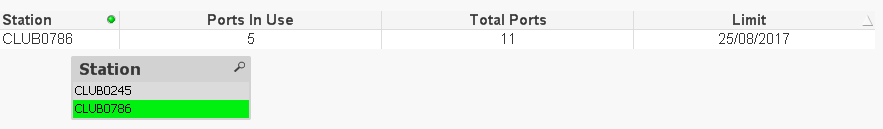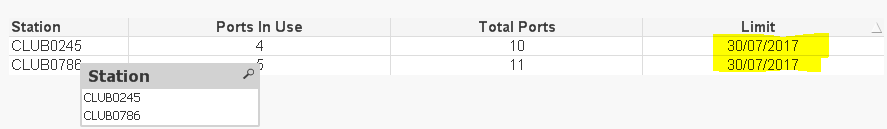# QlikView App Development

Discussion Board for collaboration related to QlikView App Development.

HighlightedPartner

## Forecast Based on trend

Hello,

I need some help with this dummy scenario. Based on the usage below and the totals I am trying to forecast the date by which the total will be hit.

any help is appreciated.

Thanks

I have attached the qvw fileTags (1)
1 Solution

Accepted SolutionsMVP

## Re: Forecast Based on trend

This may be?

Date(-LINEST_B({\$<Date=>}TOTAL <Station> If(TrendDate=Date, Aggr(Sum({\$<Date=>}[In Use]), Date,Station), Null()),

TrendDate)/LINEST_M({\$<Date=>}TOTAL <Station> If(TrendDate=Date, Aggr(Sum({\$<Date=>}[In Use]), Date,Station), Null()), TrendDate),'DD/MM/YYYY')12 RepliesMVP

## Re: Forecast Based on trend

Can you share what the expected output is and how you reach the expected output?Partner

## Re: Forecast Based on trend

Hi Synny,

am try to achieve something like.where the runt out date is forecsted based on the in use growth overtime and the total for that station.

ThanksMVP

## Re: Forecast Based on trend

Not sure I understand the logic behind 15/07/2019? Can you share an Excel file with the logic?Partner

## Re: Forecast Based on trend

Sorry Sunny, I didn't write any logic to return that date I just showing the sample output.

But what I am trying to achieve is based on a linear growth return the date the In use will Hit 10 which is the current Total.

The current total can be different for other stations Thought. But I am happy to consider the max(Total) if that will work.

ThanksPartner

## Re: Forecast Based on trend

Hi Sunny,

I have managed to put something in qlik to get the date the total limit will be hit.

my problem is that as shown on the table in the document the Date in far in the past.

is something wrong with my expression?MVP

## Re: Forecast Based on trend

May be this:

Date(-LINEST_B({\$<Date=>}TOTAL If(TrendDate=Date, Aggr(Sum({\$<Date=>}[In Use]), Date), Null()), TrendDate)/LINEST_M({\$<Date=>}TOTAL If(TrendDate=Date, Aggr(Sum({\$<Date=>}[In Use]), Date), Null()), TrendDate),'DD/MM/YYYY')Partner

## Re: Forecast Based on trend

I applied and it is setting the same date for all the stations (close to 500 in the original app) when I don't have any station selected. but when I select a station then it returns the right date for that station? I have added 1 more station to the mix as shown below .

the app is also attached.

Thank youMVP

## Re: Forecast Based on trend

This may be?

Date(-LINEST_B({\$<Date=>}TOTAL <Station> If(TrendDate=Date, Aggr(Sum({\$<Date=>}[In Use]), Date,Station), Null()),

TrendDate)/LINEST_M({\$<Date=>}TOTAL <Station> If(TrendDate=Date, Aggr(Sum({\$<Date=>}[In Use]), Date,Station), Null()), TrendDate),'DD/MM/YYYY')Partner

## Re: Forecast Based on trend

Thanks Sunny. it worked like magicnow I need to spend time and understand you expression.

Best Regards